Refer to our Texas Go Math Grade 2 Answer Key Pdf to score good marks in the exams. Test yourself by practicing the problems from Texas Go Math Grade 2 Lesson 5.2 Answer Key Make a Ten to Add.

Explore

Write the fact below the ten frame when you hear the problem that matches the model.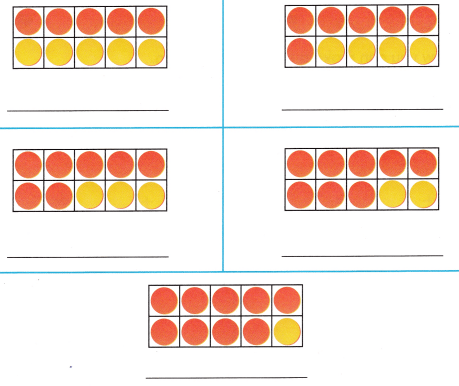Double fact method make ten strategyExplanation:
The near doubles strategy teaches the child to make connections and form relationships between facts,
as per the double fact a pair of numbers have the sum is 10, the numbers are 5 and 5.

FOR THE TEACHER • Read the following problem. There are 6 dog bones and 4 dog biscuits. How many dog treats are there? Have children find the ten frame that models the problem and write the addition sentence. Repeat by revising the story for each addition fact represented by the other ten frames.
10 dog treats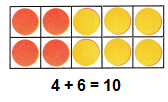Explanation:
The near doubles strategy teaches the child to make connections and form relationships between facts,
In addition if we change the order of addends there no change in sum.

Math Talk
Mathematical Processes

Describe a pattern you see in these make a ten facts.
If the set of numbers are related to each other in a specific rule, then the rule or manner is called a pattern.
Explanation:
Step 1: The first addend and what
make ten?
Step 2: Write the number below the second addend.
Step 3: The number below the second addend and what
Step 4: Add the rest to 10.
Example:
6 + 7 = 6 + 4 + 3 = 10 + 3 = 13

Model and Draw

7 + 5 = ?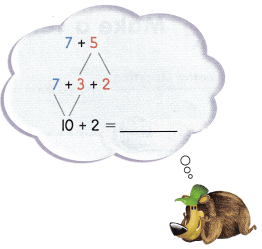You need to add 3 to 7 to make a ten. Break apart 5 as 3 and 2.
So, 7 + 5 = __________.

Share and Show

Show how you can make a ten to find the sum. Write the sum.

Question 1.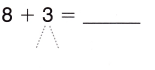10 + __________ = __________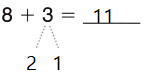10 + 1 = 11
Explanation:
8 + 3
decompose one of the addends to make a ten from the other.
So, 3 is decomposed as 2 and 1
(8 + 2) + 1 = 11
10 + 1 = 11

Question 2.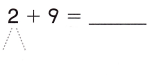10 + __________ = ___________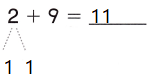10 + 1 = 11
Explanation:
2 + 9
decompose one of the addends to make a ten from the other.
So, 2 is decomposed as 1 and 1
(9 + 1) + 1 = 11
10 + 1 = 11

Question 3.
8 + 4 = ___________
10 + ___________ = ___________10 + 2 = 12
Explanation:
8 + 4
decompose one of the addends to make a ten from the other.
So, 4 is decomposed as 2 and 2
(8 + 2) + 2 = 12
10 + 2 = 12

Question 4.
4 + 7 = ___________
10 + ___________ = ___________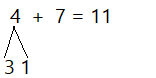10 + 1 = 11
Explanation:
4 + 7
decompose one of the addends to make a ten from the other.
So, 4 is decomposed as 3 and 1
(7 + 3) + 1 = 11
10 + 1 = 11

Question 5.
3 + 9 = ___________
10 + ___________ = ___________10 + 2 = 12
Explanation:
3 + 9
decompose one of the addends to make a ten from the other.
So, 3 is decomposed as 2 and 1
(9 + 1) + 2 = 12
10 + 2 = 12

Question 6.
7 + 6 = ___________
10 + ___________ = ___________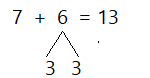10 + 3 = 13
Explanation:
7 + 6
decompose one of the addends to make a ten from the other.
So, 6 is decomposed as 3 and 3
(7 + 3) + 3 = 13
10 + 3 = 13

Problem Solving

Make a ten to find the sum. Write the sum.

Question 7.
4 + 9 = ___________
10 + ___________ = ___________
4 + 9 = 13
10 + 3 = 13
Explanation:
4 + 9
decompose one of the addends to make a ten from the other.
So, 4 is decomposed as 1 and 3
(9 + 1) + 3 = 13
10 + 3 = 13

Question 8.
9 + 8 = ___________
10 + ___________ = ___________
9 + 8 = 17
10 + 7 = 17
Explanation:
9 + 8
decompose one of the addends to make a ten from the other.
So, 8 is decomposed as 1 and 7
(9 + 1) + 7 = 17
10 + 7 = 17

Question 9.
8 + 6 = ___________
10 + ___________ = ___________
8 + 6 = 14
10 + 4 = 14
Explanation:
8 + 6
decompose one of the addends to make a ten from the other.
So, 6 is decomposed as 2 and 4
(8 + 2) + 4 = 14
10 + 4 = 14

Question 10.
5 + 9 = ___________
10 + ___________ = ___________
5 + 9 = 14
10 + 4 = 14
Explanation:
5 + 9
decompose one of the addends to make a ten from the other.
So, 5 is decomposed as 1 and 4
(9 + 1) + 4 = 14
10 + 4 = 14

Question 11.
9 + 9 = ___________
10 + ___________ = ___________
9 + 9 = 18
10 + 8 = 18
Explanation:
9 + 9
decompose one of the addends to make a ten from the other.
So, 9 is decomposed as 1 and 8
(9 + 1) + 8 = 18
10 + 8 = 18

Question 12.
8 + 4 = ___________
10 + ___________ = ___________
8 + 4 = 12
10 + 2 = 12
Explanation:
8 + 4
decompose one of the addends to make a ten from the other.
So, 4 is decomposed as 2 and 2
(8 + 2) + 2 = 12
10 + 2 = 12

Solve. Write or draw to explain.

Question 13.
H.O.T. There were 5 bees in a hive. How many more bees need to go in the hive for there to be 14 bees?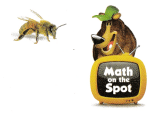__________ more bees
9 more beesExplanation:
The near doubles strategy teaches the child to make connections and form relationships between facts,
In addition if we change the order of addends there no change in sum.
9 + 5 = 14
5 + 5 + 4 = 14

Question 14.
H.O.T. Multi-Step Max is thinking of a doubles fact. The sum is greater than the sum of 6 + 4 but less than the sum of 8 + 5. What fact is Max thinking of?
_________ + __________ = __________
6 + 6 = 12
Explanation:
The sum is greater than the sum of 6 + 4 but less than the sum of 8 + 5.
6 + 4 = 10
8 + 5 = 13
12 can be written as double fact 6 + 6 =12

Question 15.
Multi-Step Peter and his dad played a game of Ball Toss. Peter scored 8 points and 7 points. His dad scored 9 points and 5 points. Who scored more points?
(A) Peter
(C) They scored the same number of points.
Option(A)
Explanation:
Peter scored 8 points and 7 points.
Total points 8 + 7 = 15
His dad scored 9 points and 5 points.
Total points 9 + 5 = 14
When compared both the points Peter scored more points.
Question 16.
There were 9 girls and 7 boys standing in line to play a game. How many children were standing in line?
(A) 15
(B) 13
(C) 16
Option(C)
Explanation:
There were 9 girls and 7 boys standing in line to play a game.
Number of children standing in line 9 + 7 = 16

Question 17.
Connect Winston has 8 tokens and Shelly has 5 tokens. Which of the following could you use to find how many tokens they have?
(A) 10 + 4
(B) 10 + 3
(C) 10 + 7
Option(B)
Explanation:
Winston has 8 tokens and
Shelly has 5 tokens.
decompose one of the addends to make a ten from the other.
So, 5 is decomposed as 2 and 3
(8 + 2) + 3 = 13
10 + 3 = 13

Question 18.
TEXAS Test Prep Natasha had 8 shells. Then she found 5 more shells. How many shells does she have now?(A) 12
(B) 13
(C) 3
Option(B)
Explanation:
Then she found 5 more shells.
Total shells 8 + 5 = 13

TAKE HOME ACTIVITY • Ask your child to name pairs of numbers that have sums of 10. Then have him or her write the addition sentences.
5 + 5 = 10
Explanation:
The near doubles strategy teaches the child to make connections and form relationships between facts,
as per the double fact a pair of numbers have the sum is 10, the numbers are 5 and 5
5 + 5 = 10

### Texas Go Math Grade 2 Lesson 5.2 Homework and Practice Answer Key

Make a ten to find the sum. Write the sum.

Question 1.
7 + 7 = ___________
10 + ___________ = ___________
7 + 7 = 14
10 + 4 = 14
Explanation:
7 + 7 = 14
decompose one of the addends to make a ten from the other.
So, 7 is decomposed as 3 + 4
(7 + 3) + 4 = 14
10 + 4 = 14

Question 2.
9 + 7 = ___________
10 + ___________ = ___________
9 + 7 = 16
10 + 6 = 16
Explanation:
9 + 7 = 16
decompose one of the addends to make a ten from the other.
So, 7 is decomposed as 1 + 6
(9 + 1) + 6 = 16
10 + 6 = 16

Question 3.
6 + 7 = ___________
10 + ___________ = ___________
6 + 7 = 13
10 + 3 = 13
Explanation:
6 + 7 = 13
decompose one of the addends to make a ten from the other.
So, 7 is decomposed as 4 + 3
(7 + 4) + 3 = 13
10 + 3 = 13

Question 4.
8 + 9 = ___________
10 + ___________ = ___________
8 + 9 = 17
10 + 7 = 17
Explanation:
8 + 9 = 17
decompose one of the addends to make a ten from the other.
So, 9 is decomposed as 2 + 7
(8 + 2) + 7 = 17
10 + 7 = 17

Problem Solving

Solve. Write or draw to explain.

Question 5.
How many more frogs are needed for there to be 13 frogs?__________ more frogs
6 more frogs.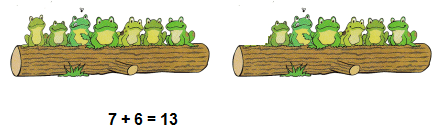Explanation:
as per the double fact method 6 + 6 = 12
for total 13
12 + 1 = 13
Question 6.
Multi-Step Alex is thinking of a doubles fact. The sum is greater than the sum of 7 + 7 but less than the sum of 8 + 9. What fact is Alex thinking of?
_________ + _________ = ___________
8 + 8 = 16
Explanation:
The sum is greater than the sum of 7 + 7 but less than the sum of 8 + 9.
7 + 7 = 14
8 + 9 = 17
16 can be written as double fact 8 + 8 = 16

Lesson Check

Question 7.
Leon saw 5 birds in the morning. Then he saw 6 more birds in the afternoon. How many birds did he see in all?
(A) 11
(B) 13
(C) 1
Option(A)
Explanation:
Leon saw 5 birds in the morning.
Then he saw 6 more birds in the afternoon.
Total birds he see in all 5 + 6 = 11

Question 8.
There were 9 girls and 8 boys waiting for the school bus. How many children were waiting for the school bus?
(A) 19
(B) 17
(C) 15
Option(B)
Explanation:
There were 9 girls and 8 boys waiting for the school bus.
Total children waiting for the school bus were 9 + 8 = 17

Question 9.
Shirley has 6 counters and Sam has 8 counters to play a game. Which could you use to find how many counters they have?
(A) 10 + 7
(B) 10 + 5
(C) 10 + 4
Option(C)
Explanation:
Shirley has 6 counters and
Sam has 8 counters to play a game.
Number of counters they have 6 + 8 = 14
decompose one of the addends to make a ten from the other.
So, 6 is decomposed as 2 + 4
(8 + 2) + 4 = 14
10 + 4 = 14

Question 10.
Multi-Step Brenda and her sister played a game. In one game, Brenda scored 6 points and then 9 points. Her sister scored 8 points and then 7 points. Who scored more points?
(A) Brenda’s sister
(B) They scored the same number of points.
(C) Brenda